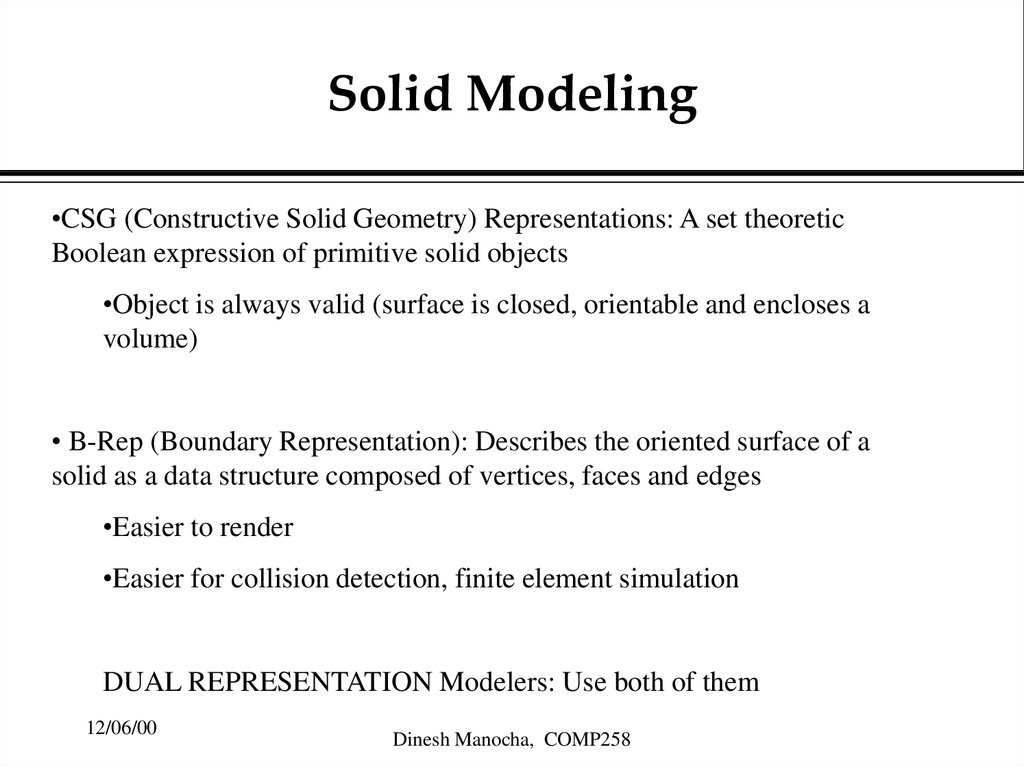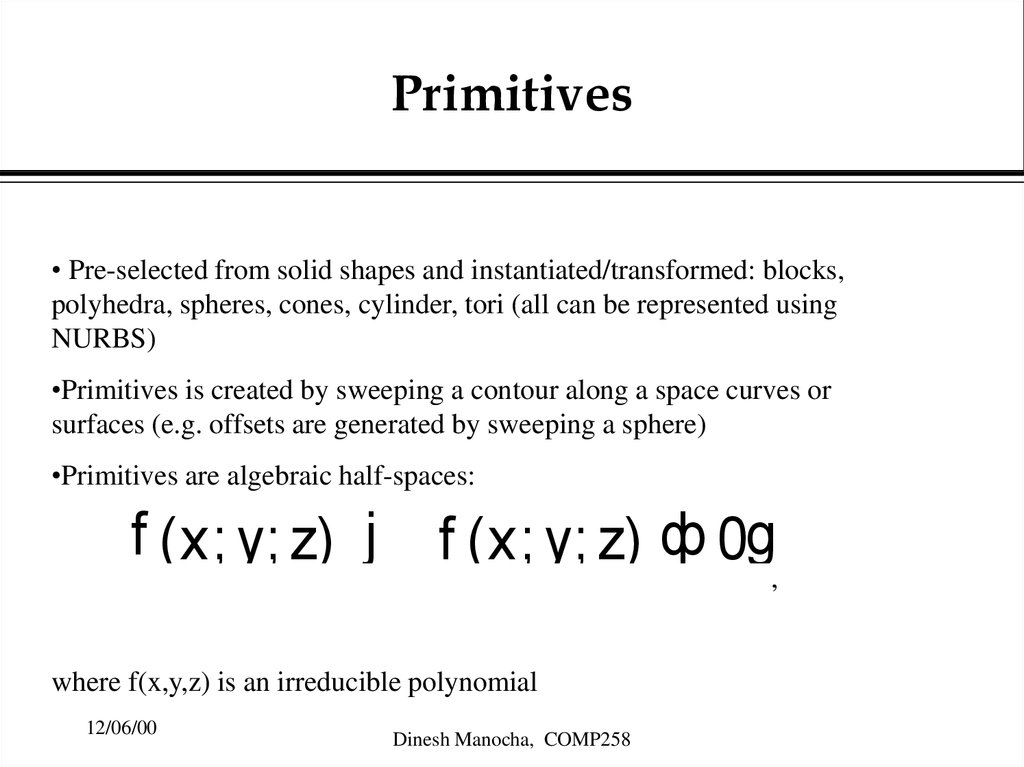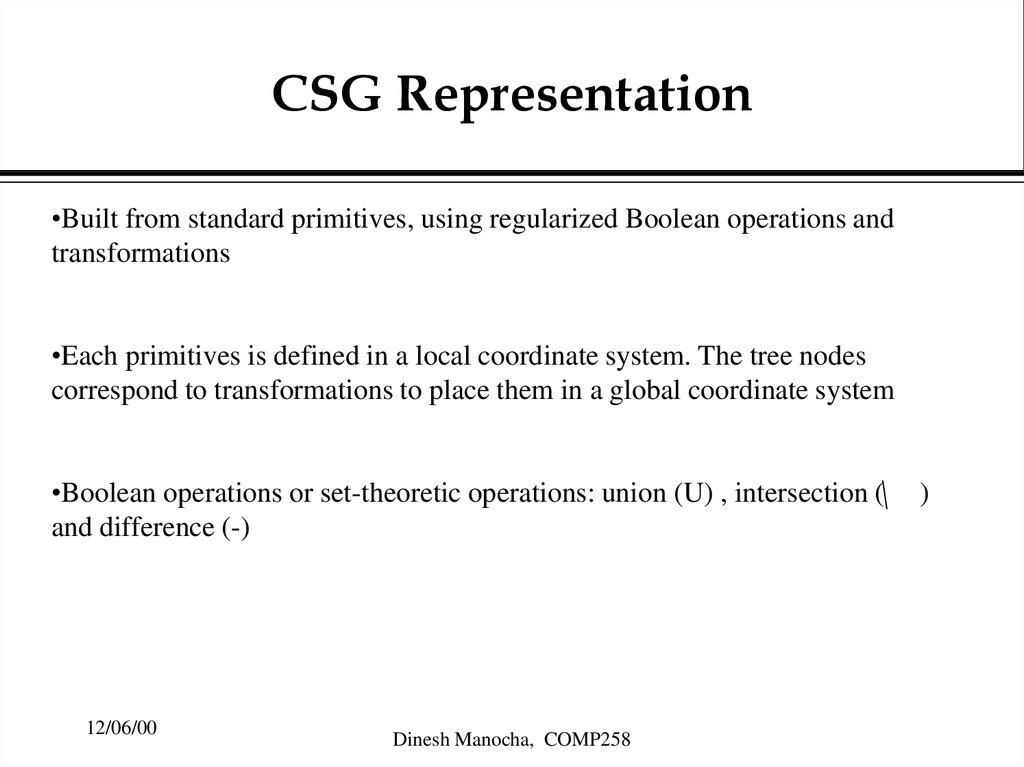# Solid Modeling

## 1. Solid Modeling

•CSG (Constructive Solid Geometry) Representations: A set theoretic
Boolean expression of primitive solid objects
•Object is always valid (surface is closed, orientable and encloses a
volume)
à
à Representation): Describes the oriented surface of a
• B-Rep (Boundary
solid as a data structure composed of vertices, faces and edges
•Easier to render
•Easier for collision detection, finite element simulation
DUAL REPRESENTATION Modelers: Use both of them
12/06/00
Dinesh Manocha, COMP258

## 2. Primitives

• Pre-selected from solid shapes and instantiated/transformed: blocks,
polyhedra, spheres, cones, cylinder, tori (all can be represented using
NURBS)
à
•Primitives is created by sweeping a contour along a space curves or
surfaces (e.g. offsets are generated by sweeping a sphere)
à
•Primitives are algebraic half-spaces:
f (x; y; z) j
f (x; y; z) ф 0g,
where f(x,y,z) is an irreducible polynomial
12/06/00
Dinesh Manocha, COMP258

## 3.

CSG Representation
•Built from standard primitives, using regularized Boolean operations and
transformations
à
•Each primitives
is defined in a local coordinate system. The tree nodes
correspond to transformations to place them in a global coordinate system
à
•Boolean operations or set-theoretic operations: union (U) , intersection (\
and difference (-)
12/06/00
Dinesh Manocha, COMP258
)

## 4. Regularized Boolean Operations

Regularized union ( [ г ), regularized intersection (\
subtraction (а г )
г
) and regularized
Difference with normal set theoretic operations: the result is the closure
of the operation on the interior of the two solids and used to eliminate
à
dangling low-dimensional
structures. To compute them:
•Compute A \
B in the set-theoretic sense.
à
•Compute the interior of A \
B (in the topological sense)
•Compute the closure the interior (i.e. all boundary points adjacent to some
interior neighborhood)
The resulting solid is the regularized intersection. In practice, they are
computed by computing A \ B and eliminate the lower-dimensional
structures.
12/06/00
Dinesh Manocha, COMP258

## 5. Point/Solid Classification

•Given a point (x,y,z), is it inside, outside or on the boundary of the solid
•Other queries include classifying a line, how a surface intersects a solid and a
test of whether two solids intersect in a non-empty volume
à
•Reduce the query to classification of the primitives of the CSG tree, involving
Downward propagation as well as upward propagation
à
•Issue: What happens when the point lies EXACTLY on the surface of the
primitive
12/06/00
Dinesh Manocha, COMP258

## 6. Tree Propagation

Downward Propagation
•If (x,y,z) arrives at a node specifying a Boolean operation, then it is passed
unchanged to the two descendants of the node
à
•If (x,y,z) arrives
at a node specifying a translation or rotation, the inverse
translation or rotation is applied to (x,y,z) and the transformed point it sent to
the node’s descendant
à
•If (x,y,z) arrives at a leaf, then the point is classified w.r.t. that primitive and
the classification (in/on/out) is returned to the parent of the leaf.
Upward Propagation
The messages contain point classification that must be combined at the
Boolean operation nodes. No work is done at nodes representing
transformations
12/06/00
Dinesh Manocha, COMP258

## 7. Neighborhoods

Problem: How to classify points that lie on the surface of a primitive solid?
Solution: Use neighborhoods. The neighborhood of a point P w.r.t. solid S, is
the intersection with S of an open ball of infinitesimal radius
centered at
P.
à
2
P is inside S, iff the neighborhood is a full ball and P is outside S, iff the
neighborhood à
is an empty ball. If P is on the surface of S, then the structure of
neighborhood depends on the local topology of S at P.
Face: Ngbd. Is a halfspace
Edge: Ngbd. Is a wedge
Vertex: Ngbd. Is a cone
12/06/00
Dinesh Manocha, COMP258

## 8. Refined Upward Propogation

Goal: Perform the respective Boolean operation on the neighborhood itself
•Account for the local geometry
•Devise suitable data structures to represent neighborhoods
à
•Transform the geometric data appropriately at the rigid motion nodes
à
In practice, involves dealing with lots of non-trivial and degenerate cases
12/06/00
Dinesh Manocha, COMP258

## 9. Curve/Solid Classification

Applications: ray tracing a CSG model; boundary evaluation
•Send the line or curve description to the leaves
à
•Partition the curve into segments labeled inside, outside, or on the surface of
the primitive
à
•Propagate the segments back upward, and merge them appropriately, by
performing Boolean operations on them
12/06/00
Dinesh Manocha, COMP258

## 10. Surface/Solid Classification

•Intersect the surface with each of the primitives from which the solid has been
constructed
à resulting curves, thereby determining the bounding edges of
•Classify the
those surface areas that are inside or outside the solid, or are on the solid’s
surface
à
•Combine the segments, appropriately oriented, constructing a boundary
representation of the respective surface areas
Surface/solid classification can be used to devise a method for converting a
CSG to B-rep model (based on generate-and-test paradigm)
12/06/00
Dinesh Manocha, COMP258

## 11.

Boundary Representations
Two parts:
•Topological description of the connectivity and orientation of vertices, edges
and faces; in terms of incidences and adjacencies
•Geometricàdescription for embedding these surface elements in space;
includes vertex positions or surface equations
à
12/06/00
Dinesh Manocha, COMP258

## 12. Manifold vs. Non-Manifold

•Manifold surface: Around every one of its points, there exists a neighborhood
that is homeomorphic to a plane: I.e. the surface can be locally deformed into a
plane without tearing it or identifying separate point with each other
•A manifold
à surface is orientable if we can distinguish two different sides, e.g.
sphere and torus
•Non-orientable
à surfaces: Moebius strip, Klein bottle
•Closed orientable manifolds partition the space into the interior, the surface
and the exterior
•A Boolean operation on two manifold objects may yield a non-manifold
results
12/06/00
Dinesh Manocha, COMP258

## 13. Common Approaches to Non-Manifold Structures

Common Approaches to NonManifold Structures
•Objects must be manifolds, so operations on solids with non-manifold results
are not allowed and are considered an error
à topological manifolds, but their embedding in 3-space permits
•Objects are
geometric coincidence of topologically separate structures (i.e. topological
interpretation is
à given to non-manifold structures); serious robustness issues;
•Non-manifold objects are permitted, both as input and as output
12/06/00
Dinesh Manocha, COMP258

## 14. Robust and Error Free Geometric Operations

•Geometric objects belong to a continuous domain, they are analyzed by
algorithms doing discrete computations (e.g. floating point numbers)
representation object
à relax the incidence tests, hard to control their implications
•Typical approach:
12/06/00
Dinesh Manocha, COMP258

## 15. Floating Point Arithmetic

•Conversion errors: Can’t represent numbers exactly using binary arithmetic
(e.g. 0.6)
•Roundoff àerrors: each arithmetic operations has roundoff error
•Catastrophic cancellation
à
12/06/00
Dinesh Manocha, COMP258

## 16. Geometric Failures due to Floating Point Arithmetic

•Computation carried out with finite precision arithmetic
•Decision tests or questions of incidence: answered by different sequence of
numerical à
computation
•Different sequences of computation are equivalent when exact arithmetic is
used, but may à
result different answers using floating point arithmetic (e.g.
incidence asymmetry checking whether intersections of lines correspond to the
same)
12/06/00
Dinesh Manocha, COMP258

## 17. Approaches for handling robustness

•Restructure the algorithm so that all interpretations of noisy numerical data
and computations are logically independent
à
•Make interdependent
logical decisions by respecting the symbolic data
exactly, but can perturb the numerical data
•When makingàinterdependent logical decisions, give priority to the numerical
data
12/06/00
Dinesh Manocha, COMP258

## 18. Specific Approaches

•Use exact arithmetic: simple for linear primitives; non-trivial for non-linear
primitives
à information (hard for non-linear primitives)
•Use symbolic
•Use perturbation approaches (limited success)
à
•General area for non-linear primitives is an open area of research
12/06/00
Dinesh Manocha, COMP258